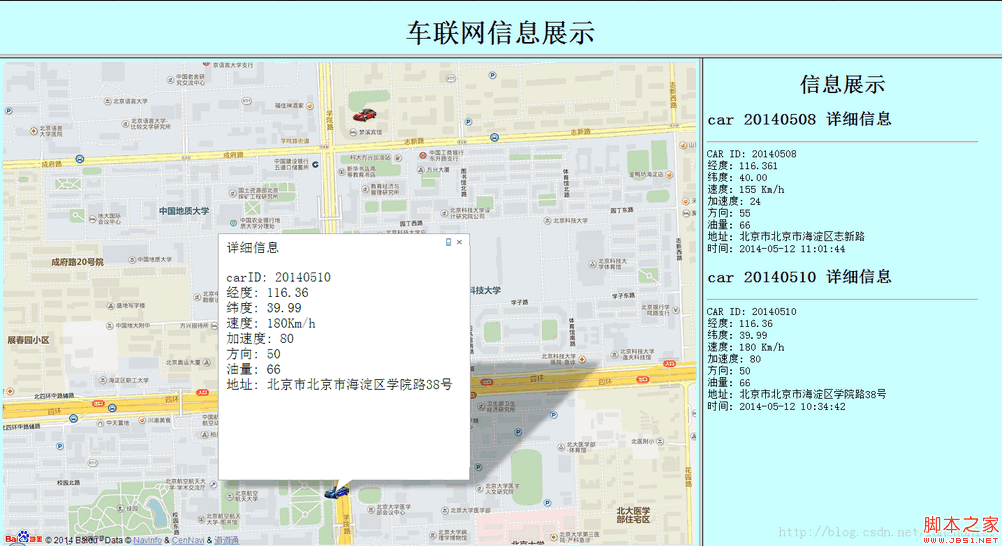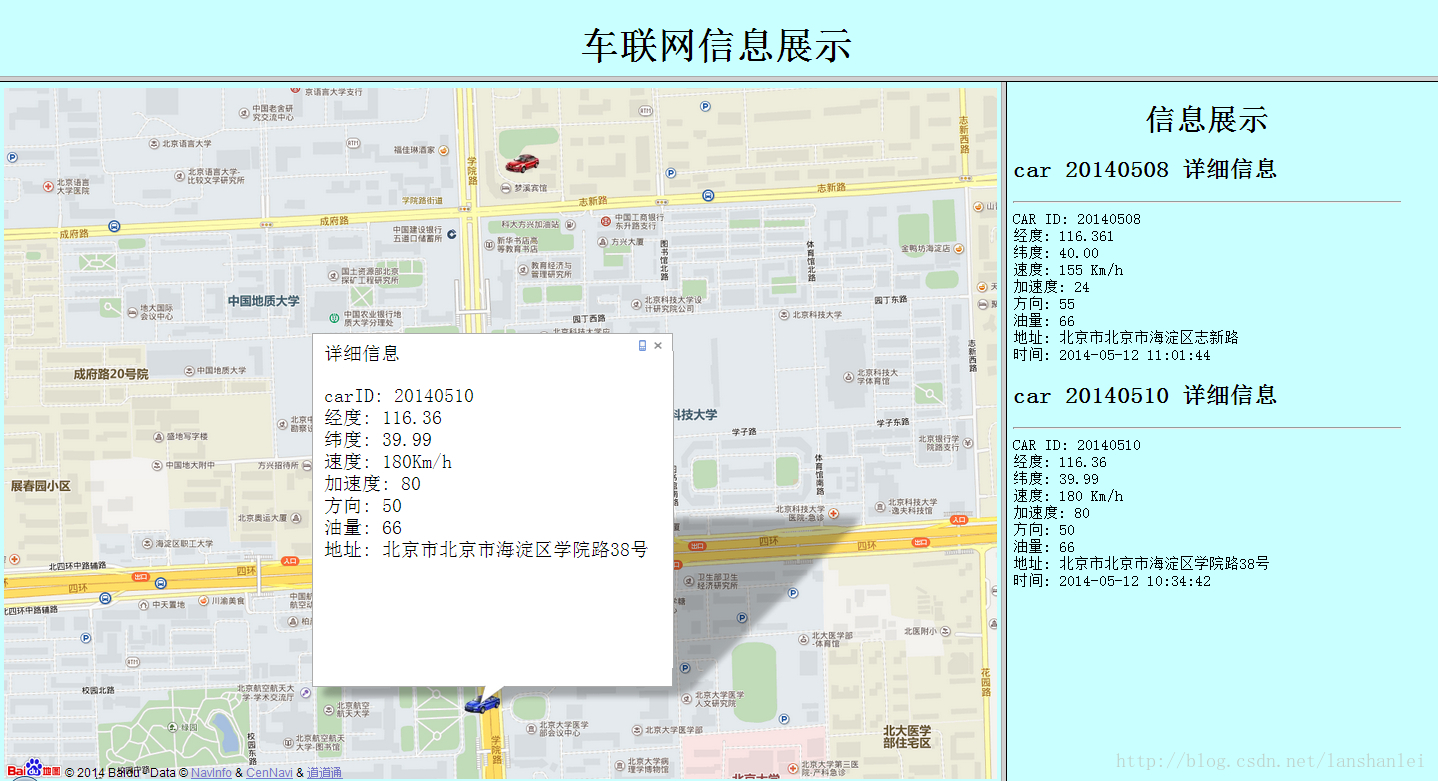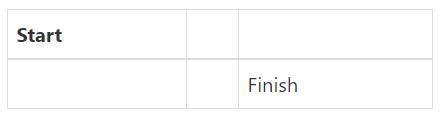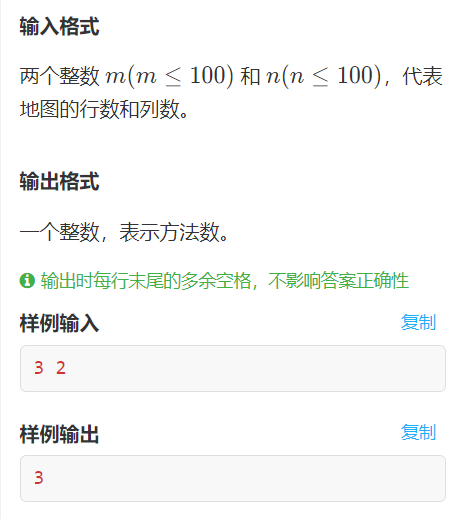•5星
842KB weixin_38037403 2021-08-26 17:48:03
•5星
49.33MB weixin_38950816 2021-04-28 18:55:32
•5星
4.68MB Mrcomj 2021-06-07 19:41:12
•5星
148KB xiaoge_586 2021-02-24 19:53:35
•5星
849KB qq_29918313 2021-07-05 11:13:55
•5星
5KB fangyango 2021-07-28 02:36:22
•5星
70.61MB zhongguonanren99 2021-06-02 14:42:52
•5星
1.89MB u013883025 2021-06-29 13:32:19
•5星
3KB qq_49747343 2020-12-04 09:11:20
•5星
62.52MB wangyue23com 2021-08-23 11:46:45
• %读入地图文件image_2=im2bw(image,0.56);%进行阈值化处理 参数可改image_d=double(image_2);obj_col=54;%设定原始列位置 可改Xobj_row=77;%设定原始行位置 可改Yinput_angle=atan(obj_row/obj_col);%设定原始速度...

image_2=im2bw(image,0.56);%进行阈值化处理 参数可改

image_d=double(image_2);

obj_col=54;%设定原始列位置 可改  X

obj_row=77;%设定原始行位置 可改  Y

input_angle=atan(obj_row/obj_col);%设定原始速度方向 可改

%-----------------------------------------

A=8;%A为分块大小 分为A*A块大小

[m,n]=size(image_d);

row=fix(m/A);%row为分块后的行数

col=fix(n/A);%col为分块后的列数

image_cell=mat2cell(image_d,ones(row,1)*A,ones(col,1)*A);

image_array=ones(A,A,row*col);

for k=1:row*col

image_array(:,:,k)=image_cell{k}(:,:);

end

%-----------------------------------------

temp_row=ceil((obj_row/A));

temp_col=ceil((obj_col/A));

number=(temp_col-1)*row+temp_row;%number为目标点所在的块的编号

%-----------------------------------------

near(1)=number-1-row;%获得邻近块编号

near(2)=number-1;

near(3)=number-1+row;

near(4)=number-row;

near(5)=number;

near(6)=number+row;

near(7)=number+1-row;

near(8)=number+1;

near(9)=number+1+row;

%-----------------------------------------

small=1000;

final_i=0;

fianl_j=0;

final_k=0;

flag=0;%标志是否找到匹配点

for k=1:9

for i=2:A-1

for j=2:A-1

if image_array(i,j,near(k))==0

temp_col_2=1+floor(near(k)/row);

temp_row_2=near(k)-(temp_col_2-1)*row;

temp_row_3=(temp_row_2-1)*A+i;

temp_col_3=(temp_col_2-1)*A+j;

dis=distance(temp_row_3,temp_col_3,obj_row,obj_col);

temp=image_array(:,:,near(k));

angle=find_near_node(temp,i,j,k,input_angle);

if deside(temp_col_3,temp_row_3,obj_col,obj_row)

all=dis*0.6+angle*0.4;

if all<=small

small=dis;

final_i=i;%最终匹配点

fianl_j=j;%最终匹配点

final_k=near(k);%最终匹配点

flag=1;

end

else

flag=0;

end

end

end

end

end

%-----------------------------------------

switch final_k

case 1

final_k=number-1-row;

case 2

final_k=number-1;

case 3

final_k=number-1+row;

case 4

final_k=number-row;

case 5

final_k=number;

case 6

final_k=number+row;

case 7

final_k=number+1-row;

case 8

final_k=number+1;

case 9

final_k=number+1+row;

end

final_col=floor(final_k/row)+1;

final_row=final_k-(final_col-1)*row;

final_x=(final_row-1)*A+final_i;

final_y=(final_col-1)*A+fianl_j;

%-----------------------------------------

imshow(image_d);

hold on

plot(obj_col,obj_row,'o')

place_x_o=num2str(obj_row);

place_y_o=num2str(obj_col);

clc;

temp=['原始点位置为',place_x_o,'行',place_y_o,'列'];

disp(temp);

if flag==1

plot(final_y,final_x,'*')

place_x=num2str(final_x);

place_y=num2str(final_y);

temp=['找到匹配点位置为',place_x,'行',place_y,'列'];

disp(temp);

else

disp('附近没有可匹配的点，请尝试修改网格大小');

end

axis([0 128 0 128]);

xlabel('.表示原始位置 *表示校准后位置');

展开全文weixin_39669982 2021-04-21 02:26:56
• 最近要做一个网页，具体内容是：上边有一个标题，下边分成两块，左边是地图。并且地图上有两个点，点击两个点有相应的提示信息，显示数据库里最新的两条数据信息。右边是一些文字说明。本人刚开始学习，做的也不是很...

最近要做一个网页，具体内容是：上边有一个标题，下边分成两块，左边是地图。并且地图上有两个点，点击两个点有相应的提示信息，显示数据库里最新的两条数据信息。右边是一些文字说明。本人刚开始学习，做的也不是很好

总体效果如下所示：首先新建map.php文件，代码如下

/*

创建与数据库的连接

*/

$conn=mysql_connect("","","") or die("can not connect to server"); mysql_select_db("hdm0410292_db",$conn);

mysql_query("set names utf8");

//选择出两辆车插入的最新数据,并将两条语句存在数组里

$sql0="select * from car_info where carID='20140508'order by id desc limit 1";$sql1="select * from car_info where carID= '20140510' order by id desc limit 1";

$sql=array($sql0,$sql1); ?> 车联网信息展示 html{ height:99%} body{ height:99.9%; width:99%; font-family:楷体_GB2312; font-size:25px} #container {height: 100%} var lon_center = 0; var lat_center = 0; var map = new BMap.Map("container"); function addMarker(point,index,s){ var fIcon = new BMap.Icon("car1.png", new BMap.Size(55, 43), { }); var sIcon = new BMap.Icon("car2.png", new BMap.Size(55, 43), { }); var myIcon = ""; // 创建标注对象并添加到地图 if(index == 20140508) myIcon=fIcon; else myIcon=sIcon; var marker = new BMap.Marker(point, {icon: myIcon}); map.addOverlay(marker); marker.addEventListener("click",function(){ var opts={width:450,height:500,title:"详细信息"}; var infoWindow = new BMap.InfoWindow(s,opts); map.openInfoWindow(infoWindow,point); }); } //遍历数组里的两条sql语句 foreach ($sql as &$value) {$query=mysql_query($value);$row=mysql_fetch_array($query); ?> var lon= <?php echo$row[longitude] ?>;

var lat= <?php echo $row[latitude] ?>; lon_center += lon; lat_center += lat; var id=<?php echo$row[id] ?>;

var info="
"+"carID: " + "<?php echo $row[carID]?>" + " " + "经度: " + "<?php echo$row[longitude]?>" + "
" +

"纬度: " + "<?php echo $row[latitude]?>" + " " + "速度: " + "<?php echo$row[speed]?>" + "Km/h"　+　"
" +

"加速度: " + "<?php echo $row[acceleration]?>" + " " + "方向: " + "<?php echo$row[direction]?>" + "
" +

"油量: " + "<?php echo $row[oil]?>" + " " + "地址: " + "<?php echo$row[street]?>";

var point = new BMap.Point(lon, lat);

addMarker(point,<?php echo $row[carID] ?>,info); } ?> var center = new BMap.Point(lon_center/2,lat_center/2); map.centerAndZoom(center, 17); map.enableScrollWheelZoom(); map.php文件主要是显示数据库里的两条信息，将这两条信息在地图上的相应的位置显示出来。 然后再建title.php,这个很简单，就是显示一个标题 信息展示 html{ height:100%;} body{ height:10%; width:99%; font-family:楷体_GB2312; font-size:25px} 信息展示 然后在建立详细信息说明模块info.php$conn=mysql_connect("","","") or die("can not connect to server");

mysql_select_db("",$conn); mysql_query("set names utf8");$sql0="select * from car_info where carID='20140508'order by id desc limit 1";

$sql1="select * from car_info where carID= '20140510' order by id desc limit 1";$sql=array($sql0,$sql1);

function htmtocode($content){$content=str_replace("\n", "
", str_replace(" ", " ", $content)); return$content;

}

?>

信息展示

html{

height:90%;}

body{

height:90%;

width:90%;

font-family:楷体_GB2312;

font-size:20px}

信息展示

$query=mysql_query($value);

$row=mysql_fetch_array($query);

?>

car <?php echo $row[carID]?> 详细信息 CAR ID: <?php echo$row[carID]?>

经度: <?php echo $row[longitude]?> 纬度: <?php echo$row[latitude]?>

速度: <?php echo $row[speed]?> Km/h 加速度: <?php echo$row[acceleration]?>

方向: <?php echo $row[direction]?> 油量: <?php echo$row[oil]?>

地址: <?php echo $row[street]?> 时间: <?php echo$row[date]?>

最后在写一个vanet.php文件，该文件主要是调用前三个文件

信息展示
展开全文weixin_29189003 2021-06-28 06:42:22
• 最近要做一个网页，具体内容是：上边有一个标题，下边分成两块，左边是地图。并且地图上有两个点，点击两个点有相应的提示信息，显示里最新的两条数据信息。右边是一些文字说明。本人刚开始学习，做的也不是很好总体...

最近要做一个网页，具体内容是：上边有一个标题，下边分成两块，左边是地图。并且地图上有两个点，点击两个点有相应的提示信息，显示里最新的两条数据信息。右边是一些文字说明。本人刚开始学习，做的也不是很好

总体效果如下所示：首先新建map.php文件，代码如下

/*

创建与数据库的连接

*/

$conn=mysql_connect("","","") or die("can not connect to server"); mysql_select_db("hdm0410292_db",$conn);

mysql_query("set names utf8");

//选择出两辆车插入的最新数据,并将两条语句存在数组里

$sql0="select * from car_info where carid='20140508'order by id desc limit 1";$sql1="select * from car_info where carid= '20140510' order by id desc limit 1";

$sql=array($sql0,$sql1); ?> 车联网信息展示 html{ height:99%} body{ height:99.9%; width:99%; font-family:楷体_gb2312; font-size:25px} #container {height: 100%} var lon_center = 0; var lat_center = 0; var map = new bmap.map("container"); function addmarker(point,index,s){ var ficon = new bmap.icon("car1.png", new bmap.size(55, 43), { }); var sicon = new bmap.icon("car2.png", new bmap.size(55, 43), { }); var myicon = ""; // 创建标注对象并添加到地图 if(index == 20140508) myicon=ficon; else myicon=sicon; var marker = new bmap.marker(point, {icon: myicon}); map.addoverlay(marker); marker.addeventlistener("click",function(){ var opts={width:450,height:500,title:"详细信息"}; var infowindow = new bmap.infowindow(s,opts); map.openinfowindow(infowindow,point); }); } //遍历数组里的两条sql语句 foreach ($sql as &$value) {$query=mysql_query($value);$row=mysql_fetch_array($query); ?> var lon= <?php echo$row[longitude] ?>;

var lat= <?php echo $row[latitude] ?>; lon_center += lon; lat_center += lat; var id=<?php echo$row[id] ?>;

var info="
"+"carid: " + "<?php echo $row[carid]?>" + " " + "经度: " + "<?php echo$row[longitude]?>" + "
" +

"纬度: " + "<?php echo $row[latitude]?>" + " " + "速度: " + "<?php echo$row[speed]?>" + "km/h"　+　"
" +

"加速度: " + "<?php echo $row[acceleration]?>" + " " + "方向: " + "<?php echo$row[direction]?>" + "
" +

"油量: " + "<?php echo $row[oil]?>" + " " + "地址: " + "<?php echo$row[street]?>";

var point = new bmap.point(lon, lat);

addmarker(point,<?php echo $row[carid] ?>,info); } ?> var center = new bmap.point(lon_center/2,lat_center/2); map.centerandzoom(center, 17); map.enablescrollwheelzoom(); map.php文件主要是显示数据库里的两条信息，将这两条信息在地图上的相应的位置显示出来。 然后再建title.php,这个很简单，就是显示一个标题 信息展示 html{ height:100%;} body{ height:10%; width:99%; font-family:楷体_gb2312; font-size:25px} 信息展示 然后在建立详细信息说明模块info.php$conn=mysql_connect("","","") or die("can not connect to server");

mysql_select_db("",$conn); mysql_query("set names utf8");$sql0="select * from car_info where carid='20140508'order by id desc limit 1";

$sql1="select * from car_info where carid= '20140510' order by id desc limit 1";$sql=array($sql0,$sql1);

function htmtocode($content){$content=str_replace("\n", "
", str_replace(" ", " ", $content)); return$content;

}

?>

信息展示

html{

height:90%;}

body{

height:90%;

width:90%;

font-family:楷体_gb2312;

font-size:20px}

信息展示

$query=mysql_query($value);

$row=mysql_fetch_array($query);

?>

car <?php echo $row[carid]?> 详细信息 car id: <?php echo$row[carid]?>

经度: <?php echo $row[longitude]?> 纬度: <?php echo$row[latitude]?>

速度: <?php echo $row[speed]?> km/h 加速度: <?php echo$row[acceleration]?>

方向: <?php echo $row[direction]?> 油量: <?php echo$row[oil]?>

地址: <?php echo $row[street]?> 时间: <?php echo$row[date]?>

最后在写一个vanet.php文件，该文件主要是调用前三个文件

信息展示

大功告成!

如您对本文有疑问或者有任何想说的，请点击进行留言回复，万千网友为您解惑！

展开全文weixin_28366053 2021-02-05 20:23:52
• 【蓝桥杯】有一张 m×n 个小方格的地图，一个机器人位于地图的左上角，它每步只能向右或者向下移动一格。... 算法 动态规划 leetcode

有一张 m×n 个小方格的地图，一个机器人位于地图的左上角（如图标记为 Start 的地方），它每步只能向右或者向下移动一格，如果走到右下角的终点（如图标记为 Finish 的地方），有多少种不同的方法？ 例如，一个 3...

题目描述

有一张 m×n 个小方格的地图，一个机器人位于地图的左上角（如图标记为 Start 的地方），它每步只能向右或者向下移动一格，如果走到右下角的终点（如图标记为 Finish 的地方），有多少种不同的方法？例如，一个 3×2 的地图，方法数是 3 种，分别是：
右 → 右 → 下
右 → 下→ 右
下 → 右 → 右最优解法：动态规划

动态规划，先初始化第一行和第一列的所有格子为1，格子内的数表示到当前格子的路径数，到第一行和第一列格子的路径只有1条，然后从第2行第2列开始，每个格子的路径数等于他上方格子的路径数加左方格子的路径数，直到填写完最后一个格子

#include<iostream>
using namespace std;
int main(){
int x,y;
cin>>x>>y;

//动态初始化二维数组
int **dp = new int*[x];
for(int i=0;i<y;i++)
{
dp[i]=new int[y];
}

//	int dp;

//将第一列初始化为 1
for(int i=0;i<x;i++)
{
dp[i]=1;
}
//将第一行初始化为 1
for(int i=0;i<y;i++)
{
dp[i]=1;
}

//从第二行第二列开始遍历,每个格子代表到达的路径数
for(int i=1;i<x;i++)
{
for(int j=1;j<y;j++)
{
dp[i][j]=dp[i][j-1]+dp[i-1][j];
}
}

cout<<dp[x-1][y-1];

}

递归求解

当x，y大于17后，递归求解方法不适用，递归函数调用消耗太多内存

#include<iostream>
using namespace std;

int f(int x,int y,int t_x,int t_y);

int main(){
int x,y;
cin>>x>>y;
int num = f(0,0,x-1,y-1);
cout<<num<<endl;
}

int f(int x,int y,int t_x,int t_y)
{
if(x>t_x||y>t_y)
return 0;
if(x==t_x||y==t_y)
return 1;
int n1 = f(x+1,y,t_x,t_y);
int n2 = f(x,y+1,t_x,t_y);
return n1+n2;
}

展开全文hhb442 2021-03-02 16:07:13
• weixin_39778668 2020-12-22 13:14:52
• weixin_34138585 2021-06-06 10:31:00
• ningxiner2016 2021-03-26 19:01:28
• 地图投影怎么做到按条件（等角、等面积、等距）投影的？ 地图上如何量方位角

weixin_35836501 2021-01-11 19:12:43
• weixin_35654096 2021-07-17 04:35:06
• Android 在百度地图上显示自己的实时位置 定位 app android

weixin_43752257 2021-01-23 10:15:41
• weixin_39719018 2021-07-27 10:33:11
• 算法自动生成迷宫地图 算法 python

weixin_51184047 2021-03-17 23:01:29
• weixin_35965587 2021-08-09 03:45:12
• 【算法 动态规划】求路径总数 ，一个机器人在m×n大小的地图的左上角（起点）。 机器人每次向下或向右移动... 算法 动态规划 java

zhangxxin 2021-04-15 17:37:40
• weixin_42365898 2021-06-12 01:45:56
• weixin_36179777 2021-01-12 02:10:59
• 野外怎样确定方位 户外辨别方向和位置的方法有哪些？ 地图上如何量方位角

weixin_39853863 2021-02-05 09:19:40
• weixin_35363895 2020-12-29 10:18:20
• weixin_34524284 2021-06-14 05:03:30
• weixin_51394621 2021-07-26 15:22:28
• 基于滑动窗口的室内三维立体栅格地图特征点提取方法与流程 立体栅格地图

weixin_32001433 2020-12-23 21:45:30
• 百度离线地图示例之十三：动态运行轨迹实现(附源码) 街景地图 离线地图 运动轨迹 vue

xiaoge_586 2021-05-12 09:05:46
• OpenDRIVE地图图形化 matlab 自动驾驶 高精地图 python

mingjiangguoyaoqin 2021-07-22 18:27:43
• ORB-SLAM3多地图管理 ORB-SLAM3

fb_941219 2021-08-17 22:14:25
• weixin_39541227 2021-06-02 20:37:07
• C语言实现推箱子游戏的地图编辑器 c语言地图编辑代码

weixin_39883906 2021-05-20 17:41:41
• weixin_32656107 2021-07-17 04:33:56
• 瓦片地图 自动驾驶

zack_liu 2021-03-04 16:24:18
• uni-app开发map地图教程 uni-app 定位 街景地图 lbs

weixin_45615467 2021-08-11 15:03:37
• 地图（Gis）中的坐标系 gis 街景地图 arcgis

weixin_42066016 2021-09-18 17:59:02...